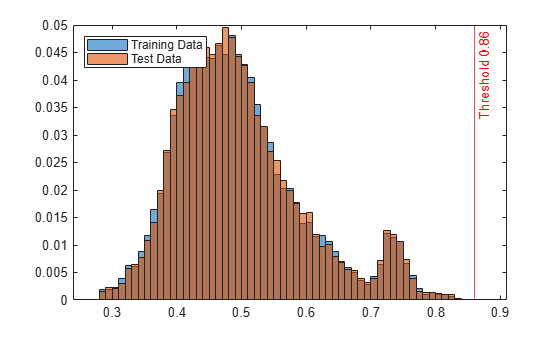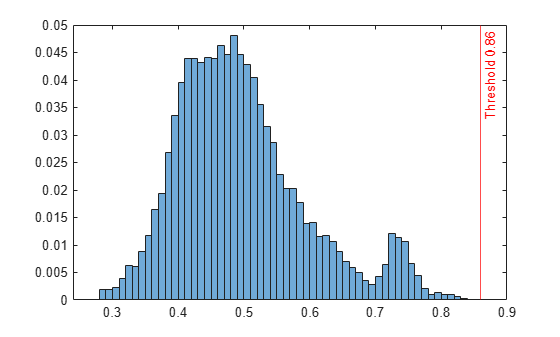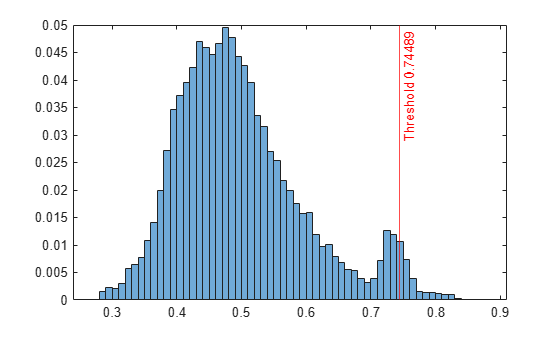isanomaly

Find anomalies in data using isolation forest

Description

example

tf = isanomaly(forest,Tbl) finds anomalies in the table Tbl using the IsolationForest object forest and returns the logical array tf, whose elements are true when an anomaly is detected in the corresponding row of Tbl. You must use this syntax if you create forest by passing a table to the iforest function.

tf = isanomaly(forest,X) finds anomalies in the matrix X. You must use this syntax if you create forest by passing a matrix to the iforest function.

example

tf = isanomaly(___,Name=Value) specifies options using one or more name-value argument combinations in addition to any of the input arguments in the previous syntaxes. For example, set ScoreThreshold=0.5 to identify observations with scores above 0.5 as anomalies.

[tf,scores] = isanomaly(___) also returns an anomaly score in the range [0,1] for each observation in Tbl or X. A score value close to 0 indicates a normal observation, and a value close to 1 indicates an anomaly.

Examples

collapse all

Create an IsolationForest object for uncontaminated training observations by using the iforest function. Then detect novelties (anomalies in new data) by passing the object and the new data to the object function isanomaly.

Load the 1994 census data stored in census1994.mat. The data set consists of demographic data from the US Census Bureau to predict whether an individual makes over \$50,000 per year.

census1994 contains the training data set adultdata and the test data set adulttest.

Train an isolation forest model for adultdata. Assume that adultdata does not contain outliers.

rng("default") % For reproducibility

Mdl is an IsolationForest object. iforest also returns the anomaly indicator tf and anomaly scores s for the training data adultdata. If you do not specify the ContaminationFraction name-value argument as a value greater than 0, then iforest treats all training observations as normal observations, that is, the values in tf are all logical 0 (false). The function sets the score threshold to the maximum score value. Display the threshold value.

Mdl.ScoreThreshold
ans = 0.8600

Use the trained isolation forest model to find anomalies in adulttest.

The isanomaly function returns anomaly indicators tf_test and scores s_test for adulttest. By default, isanomaly identifies observations with scores above the threshold (Mdl.ScoreThreshold) as anomalies.

Create histograms for anomaly scores s and s_test. Create a vertical line at the threshold of the anomaly scores.

histogram(s,Normalization="probability")
hold on
histogram(s_test,Normalization="probability")
xline(Mdl.ScoreThreshold,"r-",join(["Threshold" Mdl.ScoreThreshold]))
legend("Training Data","Test Data",Location="northwest")
hold offDisplay the observation index of the anomalies in the test data.

find(tf_test)
ans = 15655

The anomaly score distribution of the test data is similar to that of the training data, so isanomaly detects a small number of anomalies in the test data with the default threshold value. You can specify a different threshold value by using the ScoreThreshold name-value argument. For an example, see Specify Anomaly Score Threshold.

Specify the threshold value on anomaly scores by using the ScoreThreshold name-value argument of isanomaly.

Load the 1994 census data stored in census1994.mat. The data set consists of demographic data from the US Census Bureau to predict whether an individual makes over \$50,000 per year.

census1994 contains the training data set adultdata and the test data set adulttest.

Train an isolation forest model for adultdata.

rng("default") % For reproducibility

Plot a histogram of the score values. Create a vertical line at the default score threshold.

histogram(scores,Normalization="probability");
xline(Mdl.ScoreThreshold,"r-",join(["Threshold" Mdl.ScoreThreshold]))Find the anomalies in the test data using the trained isolation forest model. Use a different threshold from the default threshold value obtained when training the isolation forest model.

Determine the score threshold by using the isoutlier function.

[~,~,U] = isoutlier(scores)
U = 0.7449

Specify the ScoreThreshold name-value argument value as U.

histogram(scores_test,Normalization="probability")
xline(U,"r-",join(["Threshold" U]))Input Arguments

collapse all

Trained isolation forest model, specified as an IsolationForest object.

Predictor data, specified as a table. Each row of Tbl corresponds to one observation, and each column corresponds to one predictor variable. Multicolumn variables and cell arrays other than cell arrays of character vectors are not allowed.

If you train forest using a table, then you must provide predictor data by using Tbl, not X. All predictor variables in Tbl must have the same variable names and data types as those in the training data. However, the column order in Tbl does not need to correspond to the column order of the training data.

Data Types: table

Predictor data, specified as a numeric matrix. Each row of X corresponds to one observation, and each column corresponds to one predictor variable.

If you train forest using a matrix, then you must provide predictor data by using X, not Tbl. The variables that make up the columns of X must have the same order as the training data.

Data Types: single | double

Name-Value Arguments

Specify optional pairs of arguments as Name1=Value1,...,NameN=ValueN, where Name is the argument name and Value is the corresponding value. Name-value arguments must appear after other arguments, but the order of the pairs does not matter.

Example: ScoreThreshold=0.75,UseParallel=true sets the threshold for the anomaly score to 0.75 and instructs the function to run computations in parallel.

Threshold for the anomaly score, specified as a numeric scalar between 0 and 1. isanomaly identifies observations with scores above the threshold as anomalies.

The default value is the ScoreThreshold property value of forest.

Example: ScoreThreshold=0.5

Data Types: single | double

Flag to run in parallel, specified as true or false. If you specify UseParallel=true, the isanomaly function executes for-loop iterations in parallel by using parfor. This option requires Parallel Computing Toolbox™.

Example: UseParallel=true

Data Types: logical

Output Arguments

collapse all

Anomaly indicators, returned as a logical column vector. An element of tf is true when the observation in the corresponding row of Tbl or X is an anomaly, and false otherwise. tf has the same length as Tbl or X.

isanomaly identifies observations with scores above the threshold (the ScoreThreshold value) as anomalies.

Anomaly scores, returned as a numeric column vector whose values are between 0 and 1. scores has the same length as Tbl or X, and each element of scores contains an anomaly score for the observation in the corresponding row of Tbl or X. A score value close to 0 indicates a normal observation, and a value close to 1 indicates an anomaly.

collapse all

Isolation Forest

The isolation forest algorithm  detects anomalies by isolating anomalies from normal points using an ensemble of isolation trees.

The iforest function builds an isolation forest (ensemble of isolation trees) for training observations and detects outliers (anomalies in the training data). Each isolation tree is trained for a subset of training observations, sampled without replacements. iforest grows an isolation tree by choosing a split variable and split position at random until every observation in a subset lands in a separate leaf node. Anomalies are few and different; therefore, an anomaly lands in a separate leaf node closer to the root node and has a shorter path length (the distance from the root node to the leaf node) than normal points. The function identifies outliers using anomaly scores defined based on the average path lengths over all isolation trees.

The isanomaly function uses a trained isolation forest to detect anomalies in data. For novelty detection (detecting anomalies in new data with uncontaminated training data), you can train an isolation forest with uncontaminated training data (data with no outliers) and use it to detect anomalies in new data. For each observation of the new data, the function finds the average path length to reach a leaf node from the root node in the trained isolation forest, and returns an anomaly identifier and score.

For more details, see Anomaly Detection with Isolation Forest.

Anomaly Scores

The isolation forest algorithm computes the anomaly score s(x) of an observation x by normalizing the path length h(x):

$s\left(x\right)={2}^{-\frac{E\left[h\left(x\right)\right]}{c\left(n\right)}},$

where E[h(x)] is the average path length over all isolation trees in the isolation forest, and c(n) is the average path length of unsuccessful searches in a binary search tree of n observations.

• The score approaches 1 as E[h(x)] approaches 0. Therefore, a score value close to 1 indicates an anomaly.

• The score approaches 0 as E[h(x)] approaches n – 1. Also, the score approaches 0.5 when E[h(x)] approaches c(n). Therefore, a score value smaller than 0.5 and close to 0 indicates a normal point.

Algorithms

isanomaly considers NaN, '' (empty character vector), "" (empty string), <missing>, and <undefined> values in Tbl and NaN values in X to be missing values.

• isanomaly does not use observations with all missing values and assigns the anomaly score of 1 to the observations.

• isanomaly uses observations with some missing values to find splits on variables for which these observations have valid values.

 Liu, F. T., K. M. Ting, and Z. Zhou. "Isolation Forest," 2008 Eighth IEEE International Conference on Data Mining. Pisa, Italy, 2008, pp. 413-422.